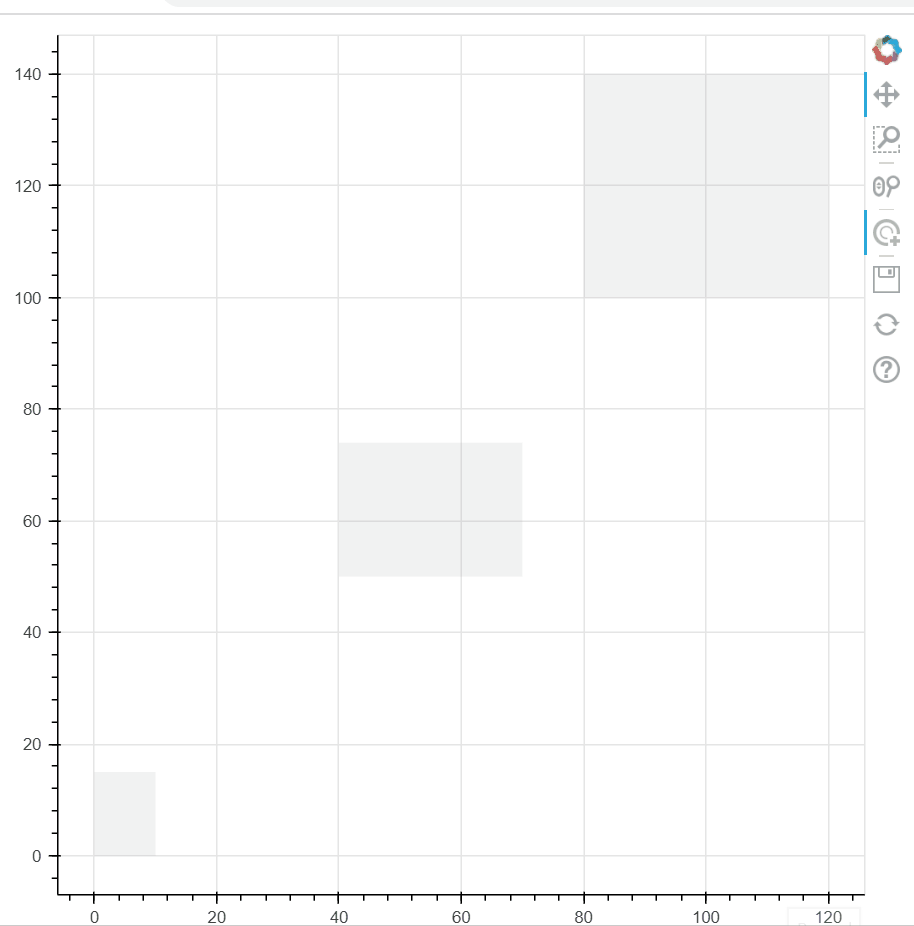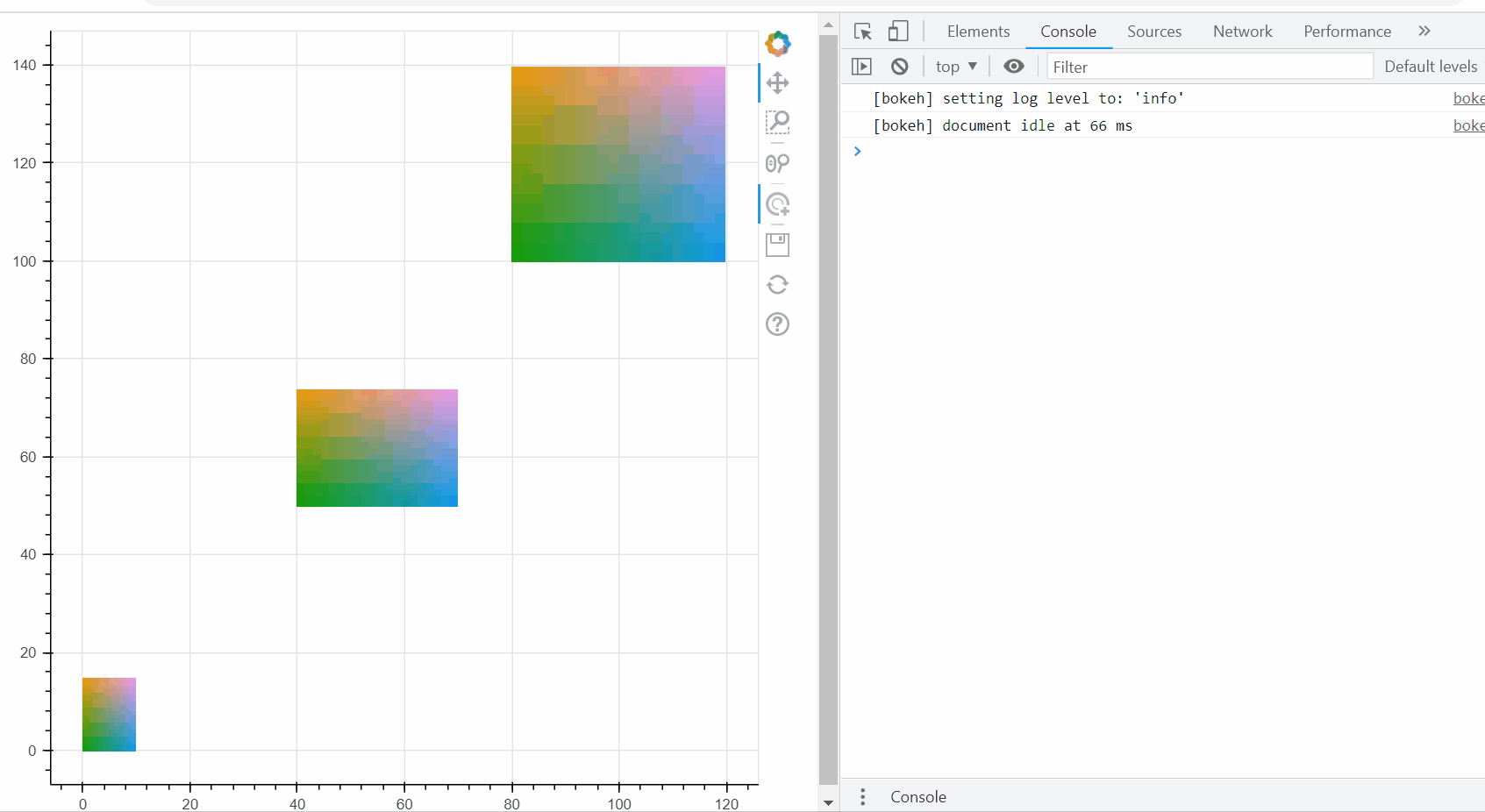# How to define the tap/selection on the image when the image is plotted by image_rgba

I am using the bokeh to implement an interactive visualization for the MNIST dataset, and the code is attached below. I am writing all the code in Jupyter Notebook following the standard practice on the official website.

``````from bokeh.plotting import figure, output_file, show
from bokeh.models import CategoricalColorMapper, ColumnDataSource, HoverTool, Column
from bokeh.io import output_notebook, show
from bokeh.plotting import figure
from bokeh import events
output_notebook()

import umap
import numpy as np

from sklearn.datasets import fetch_openml

import matplotlib.pyplot as plt
%matplotlib inline

# Encoding all the images for inclusion in a dataframe.
# reference: https://github.com/lmcinnes/umap/blob/master/doc/bokeh_digits_plot.py
# reference: https://github.com/longubu/itsne/blob/master/itsne/utils/bokeh_utils.py
from io import BytesIO
from PIL import Image
import base64

def embeddable_image(data):
img_data = 255 - 15 * data.astype(np.uint8)
image = Image.fromarray(img_data, mode='L').resize((28,28), Image.BICUBIC)
buffer = BytesIO()
image.save(buffer, format='png')
for_encoding = buffer.getvalue()
return 'data:image/png;base64,' + base64.b64encode(for_encoding).decode()

def convert_to_RGBA(img, alpha=None):
"""Converts input PIL Image or nparray to RGBA. Returns ndarray of shape
(H, W, 4), where the last channel dimension is `alpha`, the transparency"""
try:
if img.mode != "RGBA":
img = np.array(img.convert("RGBA"))
except AttributeError:
img = Image.fromarray(img)
if img.mode != "RGBA":
img = np.array(img.convert("RGBA"))
# reconvert back to npy. we converted ^ to make sure any array is converted
# to a standard color mode, RGBA, so we can manpipualate easily
if alpha is not None:
if alpha >= 0 and alpha <= 255:
img[:, :, 3] = int(alpha)
else:
raise RuntimeError("alpha must be between [0, 255]")

return np.array(img)

def preproc_img(img, flip_ud=True, alpha=None):
""" Preprocess a image arr (numpy or PIL.Image) to be absorbed bokeh's
plotting."""
# convert to RGBA if it's not, depending on whether ndarr/PIL.Image
img = convert_to_RGBA(img, alpha=alpha)

# traditional image conventions refer to top left corner as 0,0.
# however, bokeh has bottom left (0,0).
if flip_ud:
img = np.flipud(img)

# convert img to be 2d arr (M x N) with dtype = uint32 -- for bokeh
bimg = np.squeeze(img.astype(np.uint8).view(np.uint32))
return bimg

"""
Prepare the MNIST data for visualization
"""

mnist = fetch_openml('mnist_784', version=1)
mnist.target = mnist.target.astype(int)

x = mnist.data.loc[:,'pixel1':].values
y = mnist.target.loc[:].values

x_subset = x[0:1000]
y_subset = y[0:1000]

reducer = umap.UMAP(random_state=42)
embedding = reducer.fit_transform(x_subset)

x_subset_reshape = x_subset.reshape(1000,28,28)
imgs = x_subset_reshape  # make sure its numpy array for ease use

# get image shapes to convert to img object for bokeh to absorb
shape = imgs.shape
# greyscale
if len(shape) == 2:
H, W = shape
elif len(shape) == 3:
H, W, C = shape
else:
raise RuntimeError("Can't get correct image shape from first"
" image of the dataset. Got shape = %s" % shape)
bh, bw = (H / 50, W / 50)
scale = np.max([H, W]) / 5.0
xy = embedding * scale

def bkapp_plot_mnist(doc):
'''
bokeh application to plot the MNIST dataset in Jupyter Notebook
'''

x = xy[:, 0]
y = xy[:, 1]

source = ColumnDataSource(data=(dict(image = [preproc_img(imgs[i], alpha=190) for i in range(0, 1000)],
x = [x[i] - (bw / 2) for i in range(0, 1000)],
y = [y[i] - (bh / 2) for i in range(0, 1000)],
dw = [bw for i in range(0, 1000)],
dh = [bh for i in range(0, 1000)]
)
))

TOOLTIPS = [
("(x,y)", "(@x, @y)"),
]

plot_figure = figure(
title='UMAP projection of the MNIST dataset',
plot_width=800,
plot_height=800,
tools=('tap, pan, wheel_zoom, reset'),
tooltips=TOOLTIPS
)

plot_figure.image_rgba(source=source, image='image', x='x', y='y', dw='dw', dh='dh', alpha = 0.5)
plot_figure.circle(
'x',
'y',
source=source,
line_alpha=0.6,
fill_alpha=0.6,
size=4)

# define the visualizaton layout
layout = Column(plot_figure)

# show the interactive interface in the Jupyter Notebook
show(bkapp_plot_mnist)
``````

As we can see, the visualization demo can successfully plot the image via the `image_rgba` and show it on the canvas, as shown below:

By zooming in, I can successfully show the image location by moving my mouse to the image’s area and hovering there, as in the example of image ‘3’ shown below.

However, when I try to tap/click on this image, I can not select and highlight it while making other images transparent. Nothing changes, as shown below.

But when I try to click on the small blue circle on the left bottom of that image, it seems like the image can be selected because the point/circle associated with the image is being selected. The corresponding code is:

``````plot_figure.image_rgba(source=source, image='image', x='x', y='y', dw='dw', dh='dh', alpha = 0.5)
plot_figure.circle(
'x',
'y',
source=source,
line_alpha=0.6,
fill_alpha=0.6,
size=4)
``````

This is very weird at first glance, and it is inconvenient for me to interact since I need to zoom in very close to accurately click on the point/circle.

I also searched the internet and found that it seems like bokeh only defines the tap/select event for point/circle. However, this is very inconvenient for me to select the image since I may expect just to tap/click on an arbitrary pixel within the image, and then the image can be selected and highlighted.

Therefore, I want to know whether there is any way to define the tap/select event for `image_rgba`. Or are there any alternatives to do this, for example, create a rectangle that surrounds the image, and when I click on the rectangle, then I can highlight that image?

And could you explain why I can select the circle to highlight the image? What is the mechanism behind this? @Bryan, I am very interested in it since it seems like there should be a way that I can borrow some existing code in the bokeh to get the thing done.

It would also be very appreciated if you know any bokeh examples related to this issue.

Bokeh can definitely define the tap/select events for more than just point/circle. Must be an older version you are using?

I think you had a great idea with basically plotting another glyph using the same source, and selecting that instead, and since it’s the same source, you can retrieve what you need from the selected image index very easily. But yeah, is annoying if it’s a scatter/circle and having to hit the lower left perfectly. So I tried to flesh this out using a Quad, one that takes on the same dimensions of each image. The key wrinkle is that Quad takes left/right and bottom/top args, while image_rgba takes left,bottom, height and width args… but CustomJSTransform can take care of that.

I don’t have sklearn or umap in my env so I modelled this off image_rgba example on the bokeh website:

``````import numpy as np
from bokeh.plotting import figure, show
from bokeh.models import ColumnDataSource, CustomJS, Quad, CustomJSTransform,TapTool

#create a bunch of image rgbas
names = ['image1','image2','image3']
xs = [0,40,80]
ys = [0,50,100]
widths = [10,30,40]
heights= [15,24,40]
images = []
for im in range(len(xs)):
N = 20
img = np.empty((N,N), dtype=np.uint32)
view = img.view(dtype=np.uint8).reshape((N, N, 4))
for i in range(N):
for j in range(N):
view[i, j, 0] = int(i/N*255)
view[i, j, 1] = 158
view[i, j, 2] = int(j/N*255)
view[i, j, 3] = 255
images.append(img)

p = figure()

src = ColumnDataSource(data = {'x':xs,'y':ys,'widths':widths
,'heights':heights,'img':images
,'names':names})

#point image to src
#TapTool/Selection glyph fails when image is added
# imrend = p.image_rgba(image='img', x='x', y='y', dw='widths', dh='heights',source=src)
# imrend.level='underlay' #tried playing with this, didn't change anything

#also drive a quad glyph off the same source with some CustomJSTransforms
# Quad takes left, right, bottom and top args, while image_rgba basically takes left, bottom, height and width
#we can calculate the right and top args using the left, bottom, height and width fields "on the fly"
wTransform = CustomJSTransform(args=dict(src=src)
,v_func='''return xs.map((x,i)=>x+src.data['widths'][i])''') #used to add width to every x (for calculating the right)
hTransform = CustomJSTransform(args=dict(src=src)
,v_func='''return xs.map((y,i)=>y+src.data['heights'][i])''') #used to add height to every y (for calculating the top)
#create the quad glyph using these transforms
, top={'field':'y','transform':hTransform}
,fill_alpha=0.1,line_alpha=0)
#same thing but make a selection glyph
, top={'field':'y','transform':hTransform}
,fill_alpha=0.2,line_alpha=.5,fill_color='red')

tap_tool = TapTool(renderers=[qrend])

show(p)
``````

Now what’s failing seems to be a graphical bug of some sort. If I don’t render the image, I can select the quad glyph no problem:But if I uncomment the line with “imrend = …”:I’m thinking this is a bug of some sort… I tried hard coding in the right/top values for the Quad (thinking maybe it was the CustomJSTransforms) but no change in behaviour. I also tried both webgl and canvas backends, same thingI can fill out a github issue if this is indeed a bug… or am I missing something? Part of me suspects this has to do with how the image data (i.e. the ndarray) gets transformed on the BokehJS side)?

If this is just asking about “highlight” policies, I would expect this to work:

``````p.image_rgba(image='img', x='x', y='y', dw='dw', dh='dh', source=source,

# just this, like any other glyph
nonselection_alpha=0.2)
``````

The fact that it does not, I would regard as a bug. Please submit a GitHub Issue with these details.

Unfortunately I don’t have any quick or easy immediate workarounds. There is a selection made on images, but it is different than e.g. scatter (it has to be since it has to report 2D image pixel indices, etc). The design is not finalized so it has not be made publicly usable yet

This should be fixed in Bokeh 3.0 (and image selection indices made available as well):

1 Like